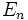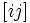# 2-cycles invariant exterior algebra

## History

### Origin

This term was introduced by: Majid

The notion of the invariant exterior algebra corresponding to the 2-cycles in the symmetric group, was first explored systematically by majod, in his paper Noncommutative differentials and Yang-Mills on S_n.

## Definition

The 2-cycles invariant exterior algebra of order$n$, denoted$\Lambda_n$, is the invariant exterior algebra defined for the symmetric group with respect to 2-cycles (or transpositions). Explicitly, it is the quotient of the free algebra on generators$e_{(ij)}$ for$1 \le i,j \le n$ by the following relations:

•$e_{(ij)} \wedge e_{(ij)} = 0$
•$e_{(ij)} \wedge e_{(km)} + e_{(km)} \wedge e_{(ij)} = 0$
•$e_{(ij)} \wedge e_{(jk)} + e_{(jk)} \wedge e_{(ki)} + e_{(ki)} \wedge e_{(ij)} = 0$

where$i,j,k,m$ are distinct.

## Parallel with the Fomin-Kirillov algebra

Further information: Fomin-Kirillov algebra

There is a close relationship between the 2-cycles invariant exterior algebra$E_n$ and the Fomin-Kirillov algebra$\Lambda_n$. In the Fomin-Kirillov algebra, the generators$[ij]$ are antisymmetric but they commute. In the 2-cycles invariant exterior algebra, the generators are symmetric and they anticommute.